# Excel VBA Multidimensional Array for Assigning Values (6 Ways)

Get FREE Advanced Excel Exercises with Solutions!

Arrays are data structures that allow you to store and manipulate large amounts of data in a single variable. They are particularly useful when dealing with large datasets or when performing calculations on multiple values at once. In this article, we’ll use Excel VBA Multidimensional Array for Assigning Values. Here we will cover the types of arrays in VBA, the procedures of assigning values to the multidimensional array, ways to reform array size, uses of multidimensional arrays, sorting array values in Excel, etc.

Want to get a quick illustration of the article we are working with? The following video will illustrate our purpose and help to get you an overview of assigning values to the multidimensional array.

## How to Launch VBA Editor in Excel

For executing VBA, you need to activate the Developer tab on the Ribbon if you haven’t done it before. After launching the Developer tab on the Home screen, launch the Visual Basic Editor window.

• Go to the Developer tab and select Visual Basic under the Code.Alternative command: Pressing ALT+F11 will also take you to the VBA window.

There are 3 ways for inserting code in the Visual Basic Editor window.

### 1. Using Module Window

• Now, the Visual Basic Editor window will show up on the screen. Click the Insert tab of the VBA window and select Module.• You will see a Module window has popped up right beside the Project – VBAProject You have to insert the code you want to execute in this window.You can either use multiple Modules for different Macros or insert your Macros one after another in the same Module. If you have different macros for serving different purposes, then it is preferable to use different Modules as it will help find your macros quickly.

Note: The code in the Module can be used for any worksheet of the workbook.

### 2. Utilizing Sheet Code Window

Besides the Module window, you can utilize the Sheet Code window.

• After opening the Visual Basic Editor, right-click on the sheet name.• From the menu, select the View Code.• You will see the Sheet (Code) window has appeared on the screen.Note: The code inserted in the sheet code window only works for that specific sheet.

### 3. Adding Button for Macro

Another way to insert VBA code is to add a Button. By adding a button and assigning Macro to that button, you can faster and automate your task.

## Introduction to Array in Excel VBA

In Excel VBA, an array is a collection of values of the same data type that are stored in a contiguous block of memory. Arrays are used to store large amounts of data in a compact and organized manner and can be useful for performing complex calculations or data manipulations.

Arrays can be categorized based on two factors.

i) By Dimensionally: Arrays can be either single-dimensional (also called one-dimensional) or multi-dimensional.

ii) By Sizing: Arrays can be either static or dynamic.

### Based on Dimension

####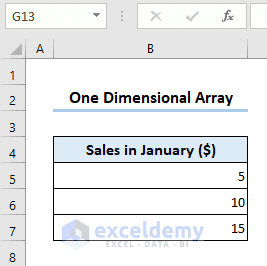In Excel VBA, a one-dimensional array is a collection of related data values stored in a single row or column of cells. It is essentially a list of values that can be accessed using a single variable name. To create a one-dimensional array in Excel VBA, you can declare it using the Dim statement, specifying the data type of the elements and the number of elements in the array.Code:

``````Sub OneDimensionalArray()
Dim Arr(1 To 3) As String
Arr(1) = 5
Arr(2) = 10
Arr(3) = 15
End Sub``````

####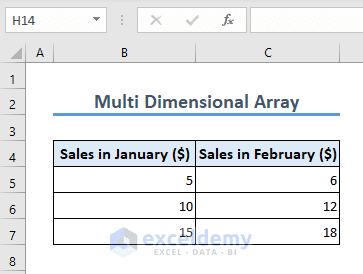In Excel VBA, a multidimensional array is a collection of related data values stored in multiple rows and columns of cells. It is essentially a table of values that can be accessed using multiple variable names.Code:

``````Sub MultiDimensionalArray()
Dim Arr(1 To 3, 1 To 3) As String
Arr(1, 1) = 5
Arr(2, 1) = 10
Arr(3, 1) = 15
Arr(1, 2) = 6
Arr(2, 2) = 12
Arr(3, 2) = 18
End Sub``````

### Based on Size

#### 1. Static Array

The default array size starts from 0. If an array with size 2 means that it can store 3 values at a time. A Static array has a fixed number of elements and a fixed size, which is determined at the time of declaration. Once the size of the array is set, it cannot be changed.The image above describes that the array has a size of 3 which means it can’t store values of more than 3.

Code:

``````Sub StaticArray()
Dim Arr(2) As Integer
End Sub``````

#### 2. Dynamic Array

A dynamic array is an array that can be resized during runtime. In contrast to a static array, where the size is determined at compile time, a dynamic array can be resized based on the current needs of the program.

To create a dynamic array in VBA, you first declare the array without specifying a size.After that, declare the array size with the ReDim statement.

Code:

``````Sub DynamicArray()
Dim Arr() As Integer
ReDim Arr(2)
End Sub``````

## How to Declare a Multidimensional Array in Excel VBA

In Excel VBA, the array is declared with the Dim command at the beginning of the subprocedure. For a multidimensional array, you have to use commas to separate each dimension.Code:

``````Sub DeclaringArray()
Dim SalesData(1 To 5, 1 To 2) As Variant
End Sub``````

This creates an array called SalesData with 5 rows and 2 columns, where each element of the array is a variant (integer or string).

## Excel VBA Multidimensional Array for Assigning Values: 6 Suitable Examples

So far you have learned about multidimensional array and the way to declare it. You can assign values to your multidimensional array based on your requirements and situations. You can assign values to your declared array, erase the value, resize the array, and also preserve the previous value after resizing the array.

###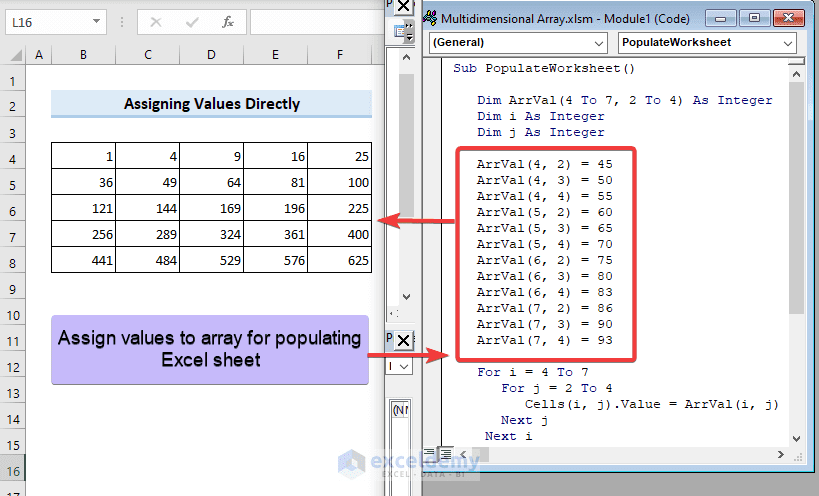You can just directly assign values to your array with the relevant dimension index and use this array to populate your Excel sheet with the array values. Here’s a sample example of populating a worksheet with array values assigned in code directly.Code:

``````Sub PopulateWorksheet()
Dim ArrVal(4 To 7, 2 To 4) As Integer
Dim i As Integer
Dim j As Integer
ArrVal(4, 2) = 45
ArrVal(4, 3) = 50
ArrVal(4, 4) = 55
ArrVal(5, 2) = 60
ArrVal(5, 3) = 65
ArrVal(5, 4) = 70
ArrVal(6, 2) = 75
ArrVal(6, 3) = 80
ArrVal(6, 4) = 83
ArrVal(7, 2) = 86
ArrVal(7, 3) = 90
ArrVal(7, 4) = 93
For i = 4 To 7
For j = 2 To 4
Cells(i, j).Value = ArrVal(i, j)
Next j
Next i
End Sub``````

###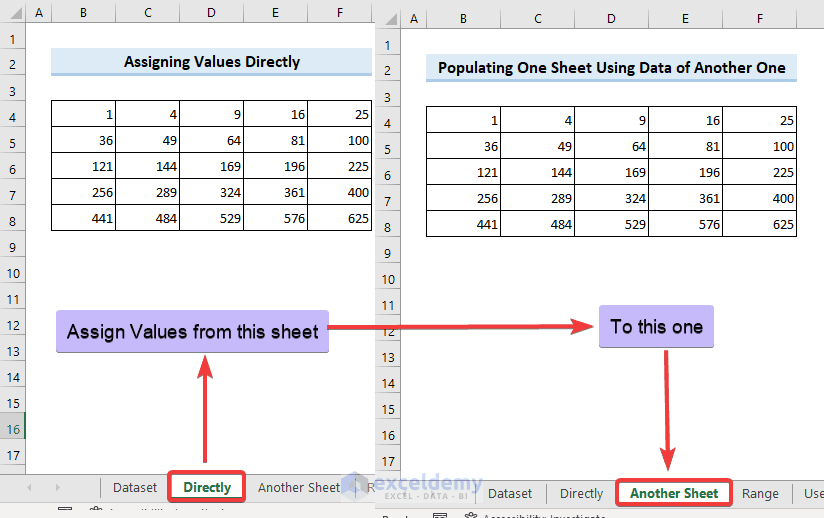When you don’t want to assign values to your array within code directly, rather you need to assign values to the array from worksheet data, in that case, this method is useful. The VBA code used here will hold the array values by reading data from one worksheet and then populate another one.Code:

``````Sub PopulatingAnotherSheet()
'Define the source worksheet and range
Dim srcWS As Worksheet
Set srcWS = ThisWorkbook.Worksheets("Directly")
Dim srcRange As Range
Set srcRange = srcWS.Range("B4:F8")
'Define the destination worksheet and range
Dim destWS As Worksheet
Set destWS = ThisWorkbook.Worksheets("Another Sheet")
Dim destRange As Range
Set destRange = destWS.Range("B4:F8")
'Copy the data to an arra
Dim dataArr() As Variant
dataArr = srcRange.Value
'Copy the array to the destination range
destRange.Value = dataArr
End Sub``````

Code Breakdown

The code copies data to array from the source range (“B4:F8”) of the source worksheet “Directly” to the destination range (“B4:F8”) of the destination worksheet “Another Sheet”.

### 3. Applying “For” Loops to Assign and Display Multidimensional Array Values

In Excel VBA, the For loop is used to iterate over a block of code a specified number of times. The For loop can be used to perform repetitive tasks, such as iterating over a range of cells, performing calculations on each cell, and storing the results in another range.

####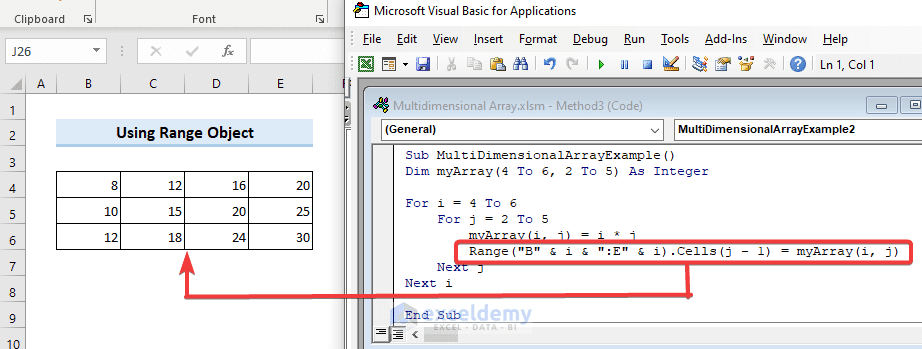The Range object in Excel VBA represents a cell or a range of cells in a worksheet. A range can be a single cell, a row, a column, or a rectangular block of cells.

Let’s see an example of a multidimensional array using the “For” loop with the “Range” object.

Here, an array will be created by multiplying the current Row and Column index number with the loop.Code:

``````Sub MultiDimensionalArrayExample()
Dim myArray(4 To 6, 2 To 5) As Integer
For i = 4 To 6
For j = 2 To 5
myArray(i, j) = i * j
Range("B" & i & ":E" & i).Cells(j - 1) = myArray(i, j)
Next j
Next i
End Sub``````

Code Breakdown

The code writes the values of the myArray to a range of cells in the worksheet. The Range object is used to specify the range of cells B4:E6 where the values should be written. The Cells property is then used to select the appropriate cell within the range, based on the current value of i and j.

The code will show the result below after executing.

#### 3.2. Utilizing UBound and LBound Functions

In Excel VBA, UBound stands for “Upper Bound” and returns the highest available index number and LBound stands for “Lower Bound” and returns the lowest available index number for a specified array dimension.

The code below utilizes VBA UBound and LBound functions and returns the same result gained with Method 3.1.Code:

``````Sub MultiDimensionalArrayExample2()
Dim myArray(4 To 6, 2 To 5) As Integer
Dim i As Long, j As Long
' Fill the array with values
For i = LBound(myArray, 1) To UBound(myArray, 1)
For j = LBound(myArray, 2) To UBound(myArray, 2)
myArray(i, j) = i * j
Next j
Next i
' Print the array values in the range "B4:E6"
For i = LBound(myArray, 1) To UBound(myArray, 1)
For j = LBound(myArray, 2) To UBound(myArray, 2)
Range("B" & i & ":E" & i).Cells(j - 1) = myArray(i, j)
Next j
Next i
End Sub``````

The code first fills the array values with the predefined size using the UBound and LBound functions and prints the array values in the range “B4:E6”. In this way, we can have VBA multidimensional array for assigning values.

### 4. Assigning New Values to 2D Dynamic Array with “ReDim” Statement

 Cake 10 Fruit 5 Drinks 20

Consider the scenario above where we have values that we can utilize for a 2 Dimensional array. Inserting the array name with a blank parenthesis is used to make the array size dynamic. Applying the ReDim statement afterward fixes the size. The ReDim statement is used to change the size of an existing dynamic array at runtime. But applying the ReDim statement changes the size of an existing array and assigns new values to the array.

Consider the following example where a dynamic array is declared with Dim Arr() syntax and the size of the array is declared 3×2 with the ReDim Arr(0 To 2, 0 To 1) statement.Code:

``````Sub ResizingArray()
Dim Arr() As String
ReDim Arr(0 To 2, 0 To 1)
Arr(0, 0) = "Cake"
Arr(0, 1) = "10"
Arr(1, 0) = "Fruit"
Arr(1, 1) = "5"
Arr(2, 0) = "Drinks"
Arr(2, 1) = "20"
MsgBox Arr(1, 0)
End Sub``````

In this code, the array size is not declared first with the Dim Arr() statement. Next the ReDim Arr(0 To 2, 0 To 1) statement is applied to define a 3×2 array, and the values are assigned to the array. You can show a random array value with MsgBox to check whether the new values are really encountered or not.ReDim statement clears the previous array and assigns a new array. If the ReDim statement is encountered again, it will clear the previous array value and create a new array. If no values are assigned again, the array will hold nothing.Code:

``````Sub ResizingArray()
Dim Arr() As String
ReDim Arr(0 To 2, 0 To 1)
Arr(0, 0) = "Cake"
Arr(0, 1) = "10"
Arr(1, 0) = "Fruit"
Arr(1, 1) = "5"
Arr(2, 0) = "Drinks"
Arr(2, 1) = "20"
'Reset the array size
ReDim Arr(0 To 3, 0 To 1)
MsgBox Arr(1, 0)
End Sub``````

This time the second ReDim will clear the value assigned with first ReDim.

###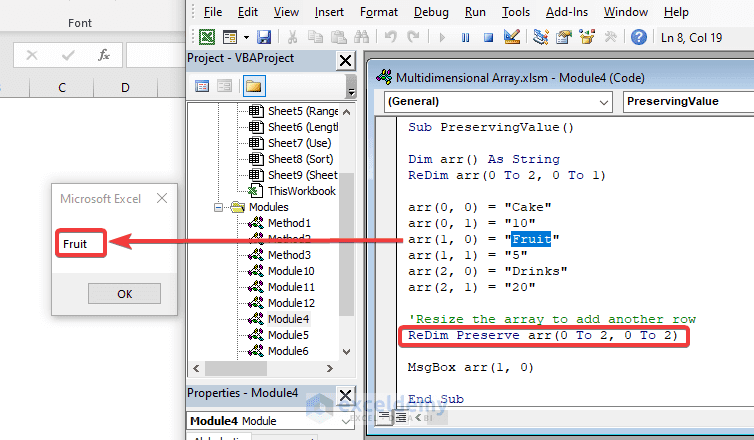The ReDim statement clears the previous array values keeping the memory blank. But sometimes you may need to increase the array size but keep the previous array values in memory. If you use the ReDim statement again, it will redefine the size as well as potentially clear array values. In that case, the ReDim Preserve statement is a lifesaver. It not only resizes the array but also keeps the previous value at the same time.Code:

``````Sub PreservingValue()
Dim Arr() As String
ReDim Arr(0 To 2, 0 To 1)
Arr(0, 0) = "Cake"
Arr(0, 1) = "10"
Arr(1, 0) = "Fruit"
Arr(1, 1) = "5"
Arr(2, 0) = "Drinks"
Arr(2, 1) = "20"
'Resize the array to add another row
ReDim Preserve Arr(0 To 2, 0 To 2)
MsgBox Arr(1, 0)
End Sub``````

Here, one thing should be kept in mind is that you can only resize the second dimension of the 2D array with ReDim Preserve. If you try to change the first dimension, it will show you a Run time error.If you had a 1-dimensional array with 10 elements, you could use Preserve to keep the first 5 elements and add 5 more elements to the end of the array. However, you have seen that this functionality is not available for multi-dimensional arrays.

To resize a multi-dimensional array while preserving the existing data, you’ll need to create a new array and copy the values from the old array to the new array. Here’s an example of how you can do this.Code:

``````Sub ResizeMultiDimArray()
Dim Arr() As String
Dim NewArr() As String
Dim i As Long, j As Long
ReDim Arr(0 To 2, 0 To 1)
Arr(0, 0) = "Cake"
Arr(0, 1) = "10"
Arr(1, 0) = "Fruit"
Arr(1, 1) = "5"
Arr(2, 0) = "Drinks"
Arr(2, 1) = "20"
'Resize the array to add another row
ReDim NewArr(0 To 3, 0 To 1)
For i = 0 To UBound(Arr, 1)
For j = 0 To UBound(Arr, 2)
NewArr(i, j) = Arr(i, j)
Next j
Next i
NewArr(3, 0) = "Snacks"
NewArr(3, 1) = "15"
MsgBox NewArr(3, 1)
End Sub``````

This will resize the first dimension and show the value in message box.

### 6. Assigning New Values to 3D Array

A 3D array in Excel VBA is a data structure that consists of three dimensions. By using a 3D array in Excel VBA, you can efficiently store and manipulate large amounts of data in a three-dimensional format.
In VBA, when you declare an array with fixed dimensions that cannot be changed afterward. However, you can modify the values of the individual elements within the array without changing the array’s size using the index number of the array element.
The code below demonstrates how to work with 3-dimensional arrays in Excel VBA and how to assign new values to specific elements of the array.Code:

``````Sub AssignNewValuesTo3DArray()
Dim Arr(0 To 2, 0 To 2, 0 To 2) As Integer
Arr(0, 0, 0) = 1
Arr(0, 0, 1) = 2
Arr(0, 0, 2) = 3
Arr(0, 1, 0) = 4
Arr(0, 1, 1) = 5
Arr(0, 1, 2) = 6
Arr(0, 2, 0) = 7
Arr(0, 2, 1) = 8
Arr(0, 2, 2) = 9
Arr(1, 0, 0) = 10
Arr(1, 0, 1) = 11
Arr(1, 0, 2) = 12
Arr(1, 1, 0) = 13
Arr(1, 1, 1) = 14
Arr(1, 1, 2) = 15
Arr(1, 2, 0) = 16
Arr(1, 2, 1) = 17
Arr(1, 2, 2) = 18
Arr(2, 0, 0) = 19
Arr(2, 0, 1) = 20
Arr(2, 0, 2) = 21
Arr(2, 1, 0) = 22
Arr(2, 1, 1) = 23
Arr(2, 1, 2) = 24
Arr(2, 2, 0) = 25
Arr(2, 2, 1) = 26
Arr(2, 2, 2) = 27
' Assign new values to the array
Arr(0, 0, 0) = 100
Arr(1, 1, 1) = 200
Arr(2, 2, 2) = 300
MsgBox Arr(1, 1, 1)
End Sub``````

After initializing the array, the code assigns new values to three specific elements of the array. The element at position (0,0,0) is assigned a value of 100, the element at position (1,1,1) is assigned a value of 200, and the element at position (2,2,2) is assigned a value of 300.

Finally, the code displays a message box showing the value of the element at position (1,1,1), which should be 200.

##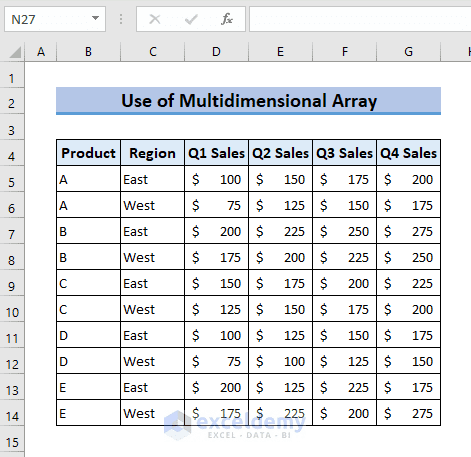From the above dataset, we will find sales for a specific Product, Region and Quarter (Q1/Q2/Q3/Q4).Code:

``````Sub GetSalesData()
Dim SalesData() As Variant
Dim Product As String
Dim Region As String
Dim Quarter As String
Dim i As Long, j As Long, k As Long
Dim Found As Boolean
SalesData = Range("B5:G14").Value ' Update range based on your dataset
' Get input values from user
Product = InputBox("Enter Product:")
Region = InputBox("Enter Region:")
Quarter = InputBox("Enter Quarter (Q1/Q2/Q3/Q4):")
' Loop through sales data to find matching values
For i = LBound(SalesData, 1) To UBound(SalesData, 1)
If SalesData(i, 1) = Product And SalesData(i, 2) = Region Then
Found = True
Select Case Quarter
Case "Q1"
MsgBox "Q1 Sales: " & SalesData(i, 3)
Case "Q2"
MsgBox "Q2 Sales: " & SalesData(i, 4)
Case "Q3"
MsgBox "Q3 Sales: " & SalesData(i, 5)
Case "Q4"
MsgBox "Q4 Sales: " & SalesData(i, 6)
Case Else
MsgBox "Invalid quarter entered."
End Select
Exit For
End If
Next i
End Sub``````

The code will take input in the input box and show output in message box.

Note: In the dataset, Product “B” is available in 2 regions: “East” and “West”. So, in the input box, we can insert a random “B” and then choose either “East” or “West”. You have no restrictions if you need to choose the region “East”, you have to select product “B” from the same row. You can choose any “Product” and select “Region” from a different row.

##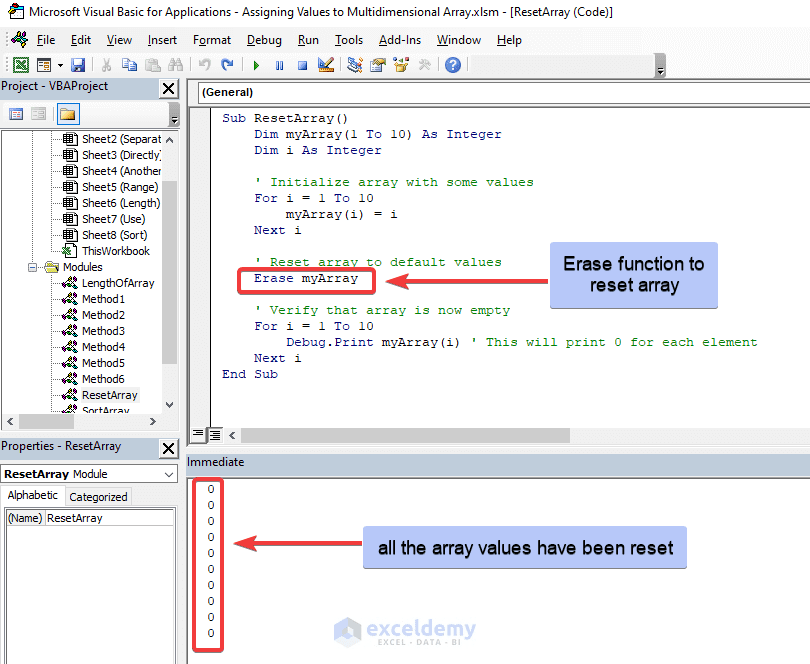The VBA Erase function clears all the array values.

Code:

``````Sub ResetArray()
Dim MyArray(1 To 10) As Integer
Dim i As Integer
' Initialize array with some values
For i = 1 To 10
MyArray(i) = i
Next i
' Reset array to default values
Erase MyArray
' Verify that array is now empty
For i = 1 To 10
Debug.Print MyArray(i) ' This will print 0 for each element
Next i
End Sub``````

## How to Get Length of Multidimensional Array in Excel VBA

There might be a situation where you will need to get the length of an array. In that case, the following code can get you on the way.Code:

``````Sub ArrayLength()
' Prompt the user to select a range on the worksheet
Dim selectedRange As Range
Set selectedRange = Application.InputBox(prompt:="Select a range to convert to array", Type:=8)
' Convert the selected range to an array
Dim selectedArray() As Variant
selectedArray = selectedRange.Value
' Get the length of the first dimension of the array
Dim length As Integer
length = UBound(selectedArray, 1) - LBound(selectedArray, 1) + 1
' Output the length to the immediate window
MsgBox "Length of selected array: " & length
End Sub``````

##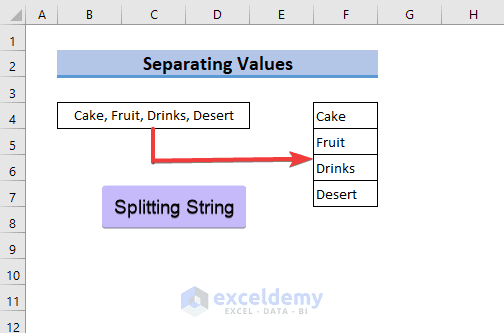When you have multiple strings in one cell and you want to split the string and put them in distinct cells, use the following VBA code.Code:

``````Sub SplittingString()
Dim inputString As String
Dim outputArray() As String
Dim i As Integer
Dim ws As Worksheet
Set ws = ThisWorkbook.Worksheets("Separate") ' Change "Sheet1" to your worksheet name
' Get the input string from cell B4
inputString = ws.Range("B4").Value
' Split the input string by comma
outputArray = Split(inputString, ",")
' Output the substrings into the range F4:F7
For i = 0 To UBound(outputArray)
ws.Cells(i + 4, 6).Value = Trim(outputArray(i))
Next i
End Sub``````

##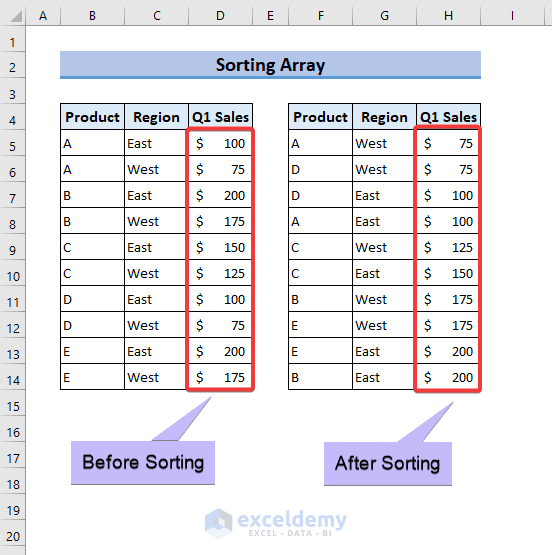When you need to take an array from a worksheet and sort the array, this method is useful in that case.Code:

``````Sub SortSales()
' Declare variables
Dim arr() As Variant
Dim i As Integer, j As Integer
Dim temp As Variant
' Get the range of data
arr = Range("B5:D14").Value
' Sort the array based on "Sales"
For i = LBound(arr, 1) To UBound(arr, 1)
For j = i + 1 To UBound(arr, 1)
If arr(j, 3) < arr(i, 3) Then
temp = arr(i, 1)
arr(i, 1) = arr(j, 1)
arr(j, 1) = temp
temp = arr(i, 2)
arr(i, 2) = arr(j, 2)
arr(j, 2) = temp
temp = arr(i, 3)
arr(i, 3) = arr(j, 3)
arr(j, 3) = temp
End If
Next j
Next i
' Output the sorted array to the worksheet
Range("F5").Resize(UBound(arr, 1), UBound(arr, 2)).Value = arr
End Sub``````

## Advantages of Using Multidimensional Array

• Multidimensional arrays allow you to organize data in a more structured and intuitive way.
• Using multidimensional arrays in Excel VBA can improve performance by reducing the number of loops and iterations in your code.
• Multidimensional arrays can simplify your code by allowing you to perform operations on multiple dimensions of data at once, rather than having to loop through multiple one-dimensional arrays.
• Multidimensional arrays in Excel VBA are highly flexible and can be used in a wide variety of applications, including data analysis, financial modeling, and simulation.

Overall, multidimensional arrays in Excel VBA provide more flexibility and organization than one-dimensional arrays, allowing you to access specific cells of the table using row and column index numbers.

• To declare a multidimensional array in VBA, you need to specify the number of dimensions and the size of each dimension.
• You can assign values to a multidimensional array in VBA using nested loops that iterate over each dimension of the array.
• You can use the LBound and UBound functions to determine the lower and upper bounds of an array.
• You can use the ReDim statement to resize a multidimensional array. When you resize an array, you can preserve the existing values by using the ReDim Preserve.

## Things to Remember

• The default array index in Excel VBA is 0 for all dimensions of the array. You can start the array index from 1 by declaring it.
• The ReDim statement only changes the second dimension of the array. If you try to change the first dimension, the code will show you a Run time error after execution.

• How do you access an element of a multidimensional array?

To access an element of a multidimensional array, you need to specify the indices for each dimension of the array. For example, if you have a two-dimensional array named “myArray” with dimensions 1 to 3 and 1 to 2, you can access the element in the second row and first column using the myArray(2,1) syntax.

• How data is stored in a multidimensional array?

In a multidimensional array, data is stored in a tabular format, where the first dimension represents the rows and the second dimension represents the columns.

## Conclusion

In this article, we have learned about Excel VBA multidimensional array for assigning values directly, populating one sheet from another, resizing size and preserving array values, and clearing array. Array has diversified use in Excel VBA. The necessity will tell you what you should use in your particular case. Don’t forget to share your thoughts in the comment box. Keep in touch with ExcelDemy.#### Rafiul Hasan

Hey there! I am Md. Rafiul Hasan. Currently, I am working as an Excel & VBA content developer. I like new ideas and want to explore the field of innovation. Excel has saved our work time and made it easy for us to perform quick calculations. I am trying to make it easier for you to overcome the obstacles you face while working on Excel. Stay connected!

We will be happy to hear your thoughtsAdvanced Excel Exercises with Solutions PDF